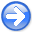PreconditioningNext: Importance of scaling Up: PRECONDITIONING THE REGULARIZATION Previous: PRECONDITIONING THE REGULARIZATION

## The second miracle of conjugate gradients

The second miracle of conjugate gradients is exhibited in the following. The data and data fitting matrix are the same, but the model damping is simplified.

 d(m)     F(m,n)                                            iter   Sum(|grad|)

-100.     62.  18.   2.  75.  99.  45.  93. -41. -15.  80.     1  69262.0000
-83.     31.  80.  92. -67.  72.  81. -41.  87. -17. -38.     2   5486.2095
20.      3. -21.  58.  38.   9.  18. -81.  22. -14.  20.     3   2755.6702
0.    100.   0.   0.   0.   0.   0.   0.   0.   0.   0.     4      0.0012
0.      0. 100.   0.   0.   0.   0.   0.   0.   0.   0.     5      0.0011
0.      0.   0. 100.   0.   0.   0.   0.   0.   0.   0.     6      0.0006
0.      0.   0.   0. 100.   0.   0.   0.   0.   0.   0.     7      0.0006
0.      0.   0.   0.   0. 100.   0.   0.   0.   0.   0.     8      0.0005
0.      0.   0.   0.   0.   0. 100.   0.   0.   0.   0.     9      0.0005
0.      0.   0.   0.   0.   0.   0. 100.   0.   0.   0.    10      0.0012
0.      0.   0.   0.   0.   0.   0.   0. 100.   0.   0.    11      0.0033
0.      0.   0.   0.   0.   0.   0.   0.   0. 100.   0.    12      0.0033
0.      0.   0.   0.   0.   0.   0.   0.   0.   0. 100.    13      0.0000


Even though the matrix is full-rank, we see the residual drop about six decimal places after the third iteration! This convergence behavior is well known in the computational mathematics literature. Despite its practical importance, it does not seem to have a name or identified discoverer. So, I call it the second miracle.''

Practitioners usually do not like the identity operator for model-space shaping. Generally, they prefer to penalize wiggliness. For practitioners, the lesson of the second miracle of conjugate gradients is that we have a choice of many iterations or learning to transform independent variables so the regularization operator becomes an identity matrix. Basically, such a transformation reduces the iteration count from something the size of the model space to something the size of the data space. Such a transformation is called preconditioning.''

More generally, the model goalintroduces a roughening operator like a gradient, a Laplacian, or in Chapter, a Prediction-Error Filter (PEF). Thus, the model goal is usually a filter, unlike the data-fitting goal that involves all manner of geometry and physics. When the model goal is a filter, its inverse is also a filter. Of course, this includes multidimensional filters with a helix.

The preconditioning transformationgives us:(8)

The operatoris a roughener, whileis a smoother. The choices of bothandare somewhat subjective. This freedom of choice suggests we eliminatealtogether by defining it to be proportional to the inverse of, thus. The fitting goals become:(9)

which enables us to benefit from the second miracle.'' After finding, we obtain the final model with.

The solutionis likely to come out smooth, because we typically over-sample axes of physical quantities. We typically penalize roughness in it by our choice of a regularization operator which means the preconditioning variabletypically has a wider frequency bandwidth than. In Chapter, we see how to make the spectrum ofcome out white (tending to flat spectrum).PreconditioningNext: Importance of scaling Up: PRECONDITIONING THE REGULARIZATION Previous: PRECONDITIONING THE REGULARIZATION

2015-05-07